# 10 Results

View
Selected filters:
• RegressionUnrestricted Use
CC BY
Rating

The book "Introductory Business Statistics" by Thomas K. Tiemann explores the basic ideas behind statistics, such as populations, samples, the difference between data and information, and most importantly sampling distributions. The author covers topics including descriptive statistics and frequency distributions, normal and t-distributions, hypothesis testing, t-tests, f-tests, analysis of variance, non-parametric tests, and regression basics. Using real-world examples throughout the text, the author hopes to help students understand how statistics works, not just how to "get the right number."

Subject:
Statistics and Probability
Material Type:
Textbook
Provider:
BCcampus
Provider Set:
BCcampus Open Textbooks
Author:
Thomas K. Tiemann
04/27/2020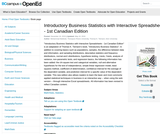Unrestricted Use
CC BY
Rating

Subject:
Statistics and Probability
Material Type:
Textbook
Provider:
BCcampus
Provider Set:
BCcampus Open Textbooks
Author:
Mohammad Mahbobi, Thompson Rivers University; Thomas K. Tiemann, Elon University
04/19/2016Unrestricted Use
CC BY
Rating

Subject:
Social Science
Material Type:
Full Course
Provider:
Rice University
Provider Set:
OpenStax College
02/14/2014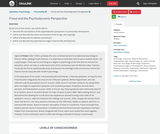Unrestricted Use
CC BY
Rating

By the end of this section, you will be able to:

Describe the assumptions of the psychodynamic perspective on personality development
Define and describe the nature and function of the id, ego, and superego
Define and describe the defense mechanisms
Define and describe the psychosexual stages of personality development

Subject:
Social Science
Material Type:
Module
Provider:
Rice University
Provider Set:
OpenStax College
06/20/2018Conditional Remix & Share Permitted
CC BY-NC-SA
Rating

This activity is an advanced version of the “Keep your eyes on the ball” activity by Bereska, et al. (1999). Students should gain experience with differentiating between independent and dependent variables, using linear regression to describe the relationship between these variables, and drawing inference about the parameters of the population regression line. Each group of students collects data on the rebound heights of a ball dropped multiple times from each of several different heights. By plotting the data, students quickly recognize the linear relationship. After obtaining the least squares estimate of the population regression line, students can set confidence intervals or test hypotheses on the parameters. Predictions of rebound length can be made for new values of the drop height as well. Data from different groups can be used to test for equality of the intercepts and slopes. By focusing on a particular drop height and multiple types of balls, one can also introduce the concept of analysis of variance.

Subject:
Statistics and Probability
Material Type:
Activity/Lab
Provider:
Provider Set:
Causeweb.org
Author:
Trent D. Buskirk and Linda J. Young, University of Nebraska-Lincoln and University of Nebraska-Lincoln
02/28/2018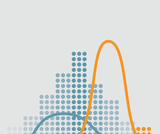Rating

Introductory statistics course developed through the Ohio Department of Higher Education OER Innovation Grant. The course is part of the Ohio Transfer Module and is also named TMM010. For more information about credit transfer between Ohio colleges and universities please visit: www.ohiohighered.org/transfer.Team LeadKameswarrao Casukhela                     Ohio State University – LimaContent ContributorsEmily Dennett                                       Central Ohio Technical CollegeSara Rollo                                            North Central State CollegeNicholas Shay                                      Central Ohio Technical CollegeChan Siriphokha                                   Clark State Community CollegeLibrarianJoy Gao                                                Ohio Wesleyan UniversityReview TeamAlice Taylor                                           University of Rio GrandeJim Cottrill                                             Ohio Dominican University

Subject:
Mathematics
Statistics and Probability
Material Type:
Full Course
Provider:
Ohio Open Ed Collaborative
04/17/2018Remix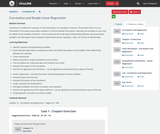Conditional Remix & Share Permitted
CC BY-NC
Rating

Sometimes it is difficult to measure or find information on a variable of interest. The problem then is to use information from easily measurable variables to find the needed information. Naturally, the variables to use must be related to the variable of interest. In this module we will study about relationships between two quantitative variables. We will explore some standard mathematical (linear, quadratic, cubic, etc.) forms of relationships.Learning Objectives:Identify response and explanatory variablesGiven bivariate data make a scatterplot of data and predict the pattern and strength of the relationship between the variablesLinear relationshipDefine correlation, study its properties and use themFind correlation for a bivariate data and interpret the resultsInterpret the square of the correlationTest for the significance of correlation – set up hypothesis and interpret the p-value of the testLinear relationship – Estimate the linear relationship between the two variables.Interpret slope and intercept.Interpret the square of the correlationStudy residuals and residual plots,Distinguish between the terms correlation and causationTest for the significance of the slope coefficient – set up hypothesis and interpret the p-value of the test.Study quadratic and other non-linear models.Textbook Material -  Chapter 12 – Correlation and Regression – Pages 673 - 699

Subject:
Statistics and Probability
Material Type:
Module
Provider:
Ohio Open Ed Collaborative
Author:
Kameswarrao Casukhela
09/06/2018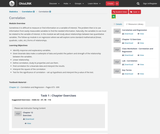Conditional Remix & Share Permitted
CC BY-NC
Rating

Sometimes it is difficult to measure or find information on a variable of interest. The problem then is to use information from easily measurable variables to find the needed information. Naturally, the variables to use must be related to the variable of interest. In this module we will study about relationships between two quantitative variables. We will explore some standard mathematical (linear, quadratic, cubic, etc.) forms of relationships.Learning Objectives:Identify response and explanatory variablesGiven bivariate data make a scatterplot of data and predict the pattern and strength of the relationship between the variablesLinear relationshipDefine correlation, study its properties and use themFind correlation for a bivariate data and interpret the resultsInterpret the square of the correlationTest for the significance of correlation – set up hypothesis and interpret the p-value of the testLinear relationship – Estimate the linear relationship between the two variables.Interpret slope and intercept.Interpret the square of the correlationStudy residuals and residual plots,Distinguish between the terms correlation and causationTest for the significance of the slope coefficient – set up hypothesis and interpret the p-value of the test.Study quadratic and other non-linear models.Textbook Material -  Chapter 12 – Correlation and Regression – Pages 673 - 699

Subject:
Statistics and Probability
Material Type:
Module
Provider:
Ohio Open Ed Collaborative